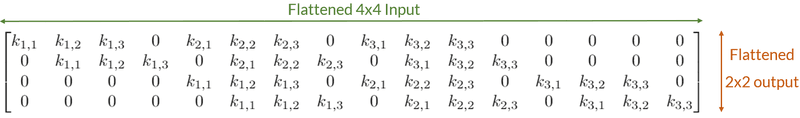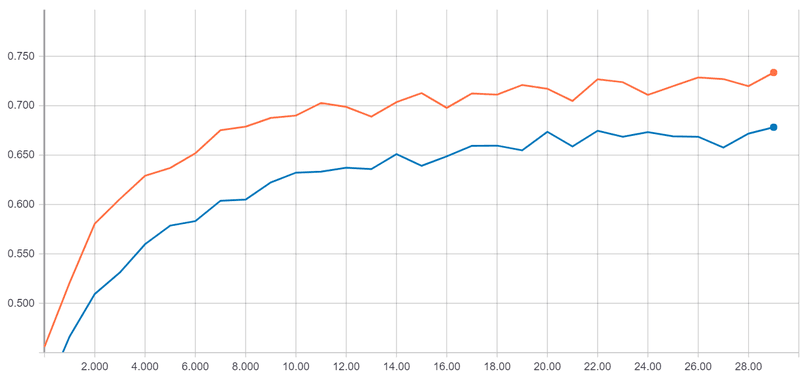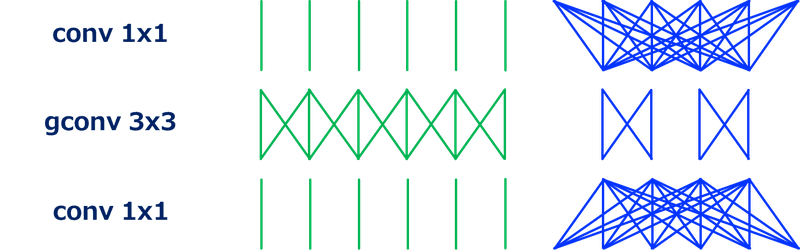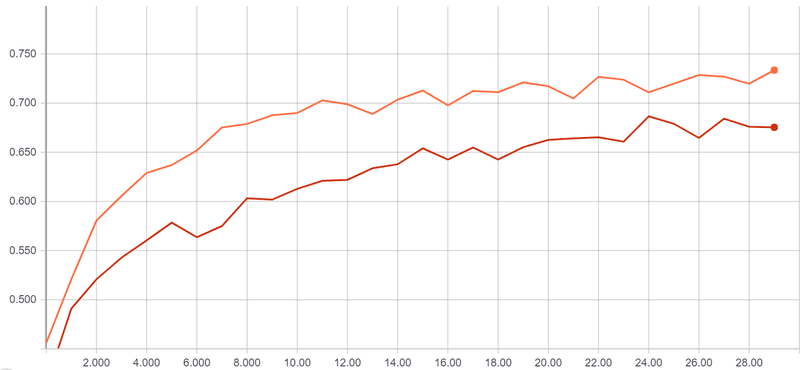# About Convolutional Layer and Convolution Kernel

Machine Learning 11 min read , December 7, 2020

A story of Convnet in machine learning from the perspective of kernel sizes.

What kernel size should I use to optimize my Convolutional layers? Let’s have a look at some convolution kernels used to improve Convnets.

Warning: This post assumes you know some basics of Machine Learning mainly with Convnets and Convolutional layers. If you don’t, check a tutorial like this one from Irhum Shafkat.

Convolutional Layers and Convnets have been around since the 1990s. They have been popularized by the ILSVRC challenge (ImageNet), a huge image recognition challenge. To win this challenge, data scientists have created a lot of different types of convolutions.

Today, I would like to tackle convolutional layers from a different perspective, which I have noticed in the ImageNet challenge. I want to focus on the kernel size and how data scientists managed to reduce the weight of their Convnets while making them deeper.

Why do weights matter? This is what we will be trying to answer first by comparing convolutional layers with fully connected ones. The next goal is tackling the question what should my kernel size be? Then we will see other convolution kernel tricks using the previously acquired ideas and how they improved Convnets and Machine Learning.

## Fully Connected vs Convolutional Layers

Convolutional layers are not better at detecting spatial features than fully connected layers. What this means is that no matter the feature a convolutional layer can learn, a fully connected layer could learn it too. In his article, Irhum Shafkat takes the example of a 4x4 to a 2x2 image with 1 channel by a fully connected layer:

We can mock a 3x3 convolution kernel with the corresponding fully connected kernel: we add equality and nullity constraints to the parameters.The fully connected equivalent of a 3x3 convolution kernel for a flattened 4x4 input and 2x2 output

The dense kernel can take the values of the 3x3 convolutional kernel.This is still the case with larger input and output vectors, and with more than one input and output channel. Even more interesting, this is the case with a 3x3, a 5x5 or any relevant kernel sizes.

Note: This requires the network inputs to be of the same size. Most Convnets use fully connected at the end anyway or have a fixed number of outputs.

So basically, a fully connected layer can do as well as any convolutional layer at any time. Well … then a fully connected layer is better than a convolutional layer at feature detection? Sadly, with neural networks, it’s not because it’s mathematically possible that it happens.

### Kernel size

A fully connected layer connects every input with every output in his kernel term. For this reason kernel size = n_inputs * n_outputs. It also adds a bias term to every output bias size = n_outputs. Usually, the bias term is a lot smaller than the kernel size so we will ignore it.

If you consider a 3D input, then the input size will be the product the width bu the height and the depth.

A convolutional layer acts as a fully connected layer between a 3D input and output. The input is the “window” of pixels with the channels as depth. This is the same with the output considered as a 1 by 1 pixel “window”.

The kernel size of a convolutional layer is k_w * k_h * c_in * c_out. Its bias term has a size of c_out.

### Fully connected layers are heavy

A “same padding” convolutional layer with a stride of 1 yields an output of the same width and height than the input. To make it simpler, let’s consider we have a squared image of size l with c channels and we want to yield an output of the same size.

So let’s take the example of a squared convolutional layer of size k. We have a kernel size of k² * c². Now if we want a fully connected layer to have the same input and output size, it will need a kernel size of (l² * c)². The kernel size ratio is l⁴ / k².

For a small image of 32x32 (MNIST, CIFAR 10) and a huge kernel size of 11x11, we are already hitting a factor of 8,600. If we got to a more realistic 224x224 image (ImageNet) and a more modest kernel size of 3x3, we are up to a factor of 270,000,000.

In the worst case, the convolutional layer is computing naively for each of the l² output pixels which gives a computing ratio of l² / k². This yields a ratio of 5,500 for the big image and small convolutional kernel and of 8.5 for the small image and the big kernel size.

We can already see that convolutional layers drastically reduce the number of weights needed. The number of weights is dependent on the kernel size instead of the input size which is really important for images. Convolutional layers reduce memory usage and compute faster.

### Overfitting

Having such a large number of parameters has another drawback: overfitting. Overfitting is when a machine learning algorithm learns too much out of the training data provided and loses the ability to generalize.

The neural network will learn different interpretations for something that is possibly the same. By forcing the shared weights among spatial dimensions, and drastically reducing the number of weights, the convolution kernel acts as a learning framework.

The same thing happens in physics. To explain the attraction or repulsion of 2 objects we used to have custom rules. Fire is going up because it was related to air. Water is going back to water … In the end, we decided that the same set of rules (gravity…) had to apply to every object and tried to find those rules and parameters out of observation. Sorry, Aristotle. By limiting the number of parameters, we are limiting the number of unrelated rules possible. This forces the machine learning algorithm to learn rules common to different situations and so to generalize better.

Convolutional layers work better than fully connected ones because they are lighter and more efficient at learning spatial features.

## Go Deeper, Not Wider

There is a general rule of thumb with neural networks. Deeper is better than wider. This is well explained in this StackExchange question. Long story short, wider networks tend to have too many weights and overfit. Whereas deeper networks will learn more interesting features: super features of the previous layer’s features.

A good way to achieve this is by making every layer lighter. This will also benefit memory usage and computational speed.

## Going Deeper, working on kernel surface

Convnets have exploded in terms of depth going from 5 or 6 layers to hundreds. Let’s talk about what happened. Remember: deeper rather than wider.

### Reducing the kernel size

Let’s look at the ILSVRC winners.

In 2012, AlexNet had a first convolution of size 11x11. In 2013, ZFNet replaced this convolutional layer by a 7x7. In 2014, GoogleNet’s biggest convolution kernel was a 5x5. It kept a first 7x7 convolutional layer. The same year, VGG, the 2nd prize, only used 3x3 convolution kernels. In the later versions, the 5x5 convolutional layer of the first version of GoogleNet has been replaced by 2 stacked 3x3 convolutional layers, copying VGG16.

What happened here? The number of parameters grows quadratically with kernel size. This makes big convolution kernels not cost efficient enough, even more, when we want a big number of channels. Remember: n = k² * c_in * c_out (kernel).

A common choice is to keep the kernel size at 3x3 or 5x5. The first convolutional layer is often kept larger. Its size is less important as there is only one first layer, and it has fewer input channels: 3, 1 by color.

### Stacking convolutions vertically

We can replace 5x5 or 7x7 convolution kernels with multiple 3x3 convolutions on top of one another.

The original throughput is kept: a block of 2 convolutional layers of kernel size 3x3 behaves as if a 5x5 convolutional window were scanning the input. (2 + 1 + 2) (5x5) is equivalent to 1 + (1 + 1 + 1) + 1 (3x3, 3x3). But it results in a lighter number of parameters: n_5x5 = 5 ² * c² > 2 * n_3x3 = 2 * 3 ² * c².  This is a ratio of 1.4 for 5x5 convolution kernel, for 7x7 convolution kernel.

Stacking smaller convolutional layers is lighter, than having bigger ones. It also tends to improve the result, with the simple intuition that it results in more layers and deeper networks.Validation Accuracy on a 3x3-based Convnet (orange) and the equivalent 5x5-based Convnet (blue)

Above is a simple example using the CIFAR10 dataset with Keras. We have 2 different Convnets. They are composed of 2 convolutions blocks and 2 dense layers. Only the construction of a block changes. In orange, the blocks are composed of 2 stacked 3x3 convolutions. In blue, the blocks are composed of a single 5x5 convolution. Notice how stacked convolutional layers yield a better result while being lighter.

## Going Further, working on kernel depth

A lot of tricks are used to reduce the convolution kernel size. They are working on the channel depth and the way the channels are connected.

### 1x1 convolutions

Convolutions layers are lighter than fully connected ones. But they still connect every input channels with every output channels for every position in the kernel windows. This is what gives the c_in * c_out multiplicative factor in the number of weights. ILSVRC’s Convnets use a lot of channels. 512 channels are used in VGG16’s convolutional layers for example.  1x1 convolution is a solution to compensate for this. It obviously doesn’t bring anything at the spatial level: the kernel acts on one pixel at a time. But it acts as a fully connected layer pixel-wise.

We would usually have a 3x3 kernel size with 256 input and output channels. Instead of this, we first do a 1x1 convolutional layer bringing the number of channels down to something like 32. Then we perform the convolution with a 3x3 kernel size. We finally make another 1x1 convolutional layer to have 256 channels again.

Wrapping a convolution between 2 convolutional layers of kernel size 1x1 is called a bottleneck. This is used in ResNet, a convnet published in 2015.

The first solution needs 3 ² 256 ²= 65,536 weights. The second one needs 1 ² 256 * 32 + 3 ² 32 ²+ ² * 32 * 256 = 25,600 weights. The convolution kernel is more than 2 times lighter.

A 1x1 convolution kernel acts as an embedding solution. It reduces the size of the input vector, the number of channels. It makes it more meaningful.The 1x1 convolutional layer is also called a Pointwise Convolution.

### Grouped convolutions or stacking convolution horizontally

Convolutional layers normally combine each input channels, to make each output channels. One can make subgroups of input channels. They will be combined independently of other groups into their output channels. This is what we call Grouped Convolution. This works as splitting the layers into subgroups, making a convolution on each of them, then stacking the output layers together.

It reduces the number of weights needed because each subgroup acts as a separated convolution of lower size. With 2 groups and 512 layers, we have 2 * k² * 256 ² instead of k² * 512 ² for a kernel size of k².Spatial (green) and layer (blue) connections in a bottleneck with grouped convolution.

This is used for example in the bottleneck of ResNeXt, a convnet published in 2015. It makes a lighter convolution kernel with more meaningful features.

### Separable convolution

When the number of groups is the number of channels, the convolution kernel doesn’t combine layers. This extreme is what we call a Depthwise Convolution. It is often combined with Pointwise Convolutional layers to uncouple spatial and layer computation.

The convolution kernel size needed for a depthwise convolutional layer is n_depthwise = c * (k² * 1 ²). Uncoupling those 2 reduces the number of weights needed: n_separable = c * (k² * 1 ²) + 1 ² * c². Considering a 5x5 convolutional layer, k² is smaller than c > 128. That let us with a ratio of approximately the kernel surface: 9 or 25. With separable convolutions, the bottleneck is in the 1x1 convolution. The equivalent separable convolutional layer is a lot lighter by approximately the convolution kernel surface.Validation Accuracy on a 3x3-based Convnet (orange) and the equivalent separable convolution-based Convnet (red)

Above is a simple example using the CIFAR10 dataset with Keras. We have 2 different Convnets. They are composed of 2 convolutions blocks and 2 dense layers. Only the construction of a block changes. In orange, the blocks are composed of 2 normal 3x3 convolutions. In red, the blocks are composed of 2 separable 3x3 convolutions. Even if the separable convolution is a bit less efficient, it is times lighter.

People have tried to go even further. They uncoupled the 2 spatial dimensions with a 3x1 kernel size followed by a 1x3 kernel size for example.

You can find a lot of similar Convnet’s strategies explained in this blog post.

Over the years, Convnets have evolved to become deeper and deeper. As layers are memory heavy and tend to overfit, a lot of strategies are created to make the convolutional layers lighter and more efficient. In general, this has been done by reducing the number of weights in one way or another.

I wanted to showcase this phenomenon in this blog post. I hoped you enjoyed. :)

Today, we’ve seen a solution to achieve the same performance as a 5x5 convolutional layer but with 13 times fewer weights.

Thanks to Dan Ringwald and Antoine Toubhans.

Related stories
Get our Battle-Tested Tutorials Delivered Straight Into Your Inbox Every Week